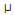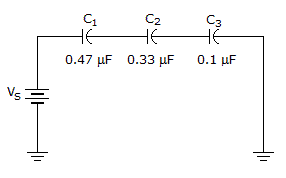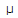# Electronics - Capacitors

### Exercise :: Capacitors - General Questions

21.

If a current of 40 mA flows through a series circuit consisting of a 0.4F capacitor and a resistor in series with a 4 kHz, 40 Vac source, what is the total circuit impedance?

 A. 10B. 100C. 1 kD. 1 MExplanation:

No answer description available for this question. Let us discuss.

22.What is the capacitance of the circuit shown in the given circuit?

 A. 0.066F B. 0.9F C. 65.97 pF D. 900 pF

Explanation:

No answer description available for this question. Let us discuss.

23.

In an ac circuit, an output capacitor and a resistor are connected in series with an ac signal. What filter action will be the result?

 A. bandpass B. low-pass C. high-pass D. band-stop

Explanation:

No answer description available for this question. Let us discuss.

24.

What is the total opposition to current in a series RC circuit called?

 A. impedance B. inductance C. reactance D. resistance

Explanation:

No answer description available for this question. Let us discuss.

25.

Which of the following statements is true?

 A. The voltage to which a capacitor is charged can change instantaneously. B. The current in a capacitive circuit takes time to change. C. A fully charged capacitor appears as a short to dc current. D. An uncharged capacitor appears as a short to an instantaneous change in current.